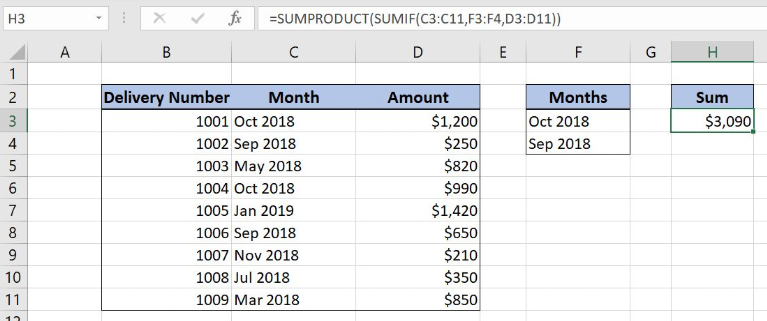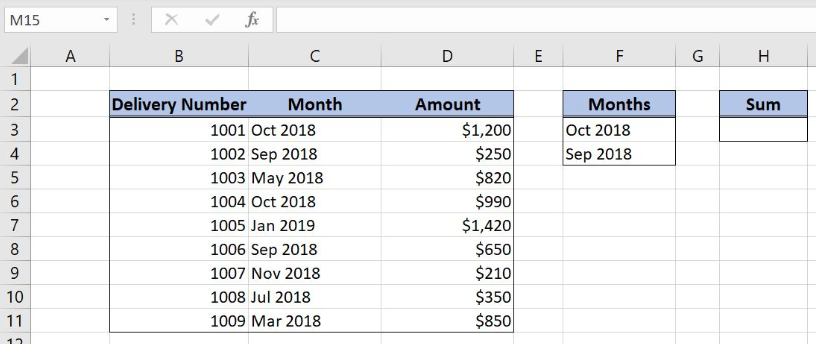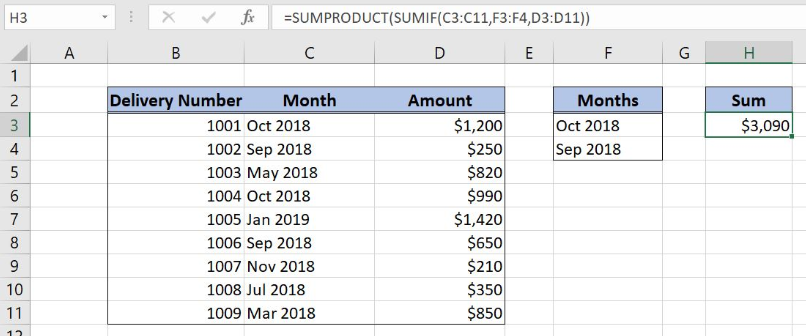Get instant live expert help with Excel or Google Sheets“My Excelchat expert helped me in less than 20 minutes, saving me what would have been 5 hours of work!”

#### Post your problem and you’ll get Expert help in seconds.

Your message must be at least 40 characters
Our professional Expert are available now. Your privacy is guaranteed.

# Sum if equal to one of many things

Excel allows a user to sum values if a cell is equal to one of many values from the other range, using the SUMPRODUCT and SUMIF functions. This step by step tutorial will assist all levels of Excel users in summing values if a cell is equal to one of many things.Figure 1. The result of the formula

## Syntax of the SUMIF Formula

The generic formula for the SUMIF function is:

`=SUMIF(range, criteria_range, [sum_range])`

The parameters of the SUMIF function are:

• range – a range where we want to apply our criteria
• criteria – a criteria range where we want to find a value from a range
• [sum_range] – a range which we want to sum. This is an optional parameter. If it’s omitted, the range will be summed.

## Syntax of the SUMPRODUCT Formula

`=SUMPRODUCT(array)`

The parameter of the SUMPRODUCT function is:

• array – an array of values which we want to sum.

## Setting up Our Data for the Formula

Let’s look at the structure of the data we will use. Our table consists of 3 columns: “Delivery Number” (column B), “Month” (column C) and “Amount” (column D). The criteria range is F3:F4 (“Months”). The result will be in the cell H3.Figure 2. Data that we will use in the example

## Sum Amounts if a Cell is Equal to One of Many Things

In our example, we want to sum all amounts which corresponding month is Oct or Sep 2018. The result is in the cell H3.

The formula looks like:

`=SUMPRODUCT(SUMIF(C3:C11, F3:F4, D3:D11))`

The range of the SUMIF function is the C3:C11 range, while the criteria is F3:F4. The sum_range parameter is D3:D11. The result of the function is the array parameter of the SUMPRODUCT function. Finally, the function sums all these values.

To apply the formula, we need to follow these steps:

• Select cell H3 and click on it
• Insert the formula: `=SUMPRODUCT(SUMIF(C3:C11, F3:F4, D3:D11))`
• Press enterFigure 3. Using the formula to sum if a cell is equal to one of many things

As we can see in Figure 3, 4 rows meet the criteria of the SUMIF function. Rows 3 and 6 have Oct 2018 and rows 4 and 8 have Sep 2018. Therefore, the result in H3 is the sum of corresponding amounts (\$1,200, \$990, \$250 and \$650) – \$3,090.

Most of the time, the problem you will need to solve will be more complex than a simple application of a formula or function. If you want to save hours of research and frustration, try our live Excelchat service! Our Excel Experts are available 24/7 to answer any Excel question you may have. We guarantee a connection within 30 seconds and a customized solution within 20 minutes.

### Did this post not answer your question? Get a solution from connecting with the expert.Another blog reader asked this question today on Excelchat:
Solution examplesamending a formula so that there is another criteria and if it does not meet this criteria then we stick with the original criteria
Solved by A. B. in 40 minsI need Column D to = Column C - Column A only if Column B has a 'Date' entered.
Solved by I. B. in 11 minsHello, I need help trying to create a sum of numerical values on a spreadsheet in which the only values that apply to the sum are when the status of another cell is a certain value (e.g. value is not in sum when status: closed, value is in sum when status: open)
Solved by T. W. in 18 minsi have a table with Dates, First names , Last names and i want to count how many times a name occurs, but if a name occurs more than once on a particular date i need to only count it once. this is on excel and not google sheets
Solved by F. H. in 31 minsI need cell B2 Green if cells G2:Q2 are empty. If cells G2:Q2 have any text in any cell then i need B2 to be red.
Solved by C. W. in 20 mins## Subscribe to Excelchat.coAnother blog reader asked this question today on Excelchat: